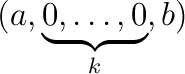## CGAL::number_of_real_roots

#include <CGAL/polynomial_utils.h>

### Definition

Given a polynomial f, or a range of values that is interpreted as the principal Sturm-Habicht coefficients of f, the function computes

 m:=# {α ∈ ℝ | f(α)=0}
that is, the number of distinct real roots of f.

The coefficient type of the polynomial, or the value type of the iterator range, respectively must be a model of RealEmbeddable. In the second version, it is not required to pass the exact princiapl Sturm-Habicht coefficients to the functions; it is only required that the sign of each element corresponds to the sign of the actual principal Sturm-Habicht coefficient.

We explain the internals of this function. For a sequence I:=(a0, ,an) of real numbers with a0 0, define

C(I)=
 s ∑ i=1
ε
i
where s is the number of subsequences of I of the formwith a 0,b 0, k 0.
For the i-th subsequence of I, define
εi:=
 0 if k is odd, (-1)k/2sign(ab) if k is even.

For f [x] with deg f=n, we have:

 C(sthan(f), … ,stha0(f)) = #{α ∈ ℝ | f(α)=0}
In other words, the signs of the principal Sturm-Habicht coefficients determine the number of distinct real roots of f.

### Operations

 template int number_of_real_roots ( Polynomial_d f) computes the number of distinct real roots of f template int number_of_real_roots ( InputIterator start, InputIterator end) computes the number of distinct real roots of f whose principal Sturm-Habicht coefficients are passed by the iterator range.